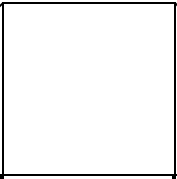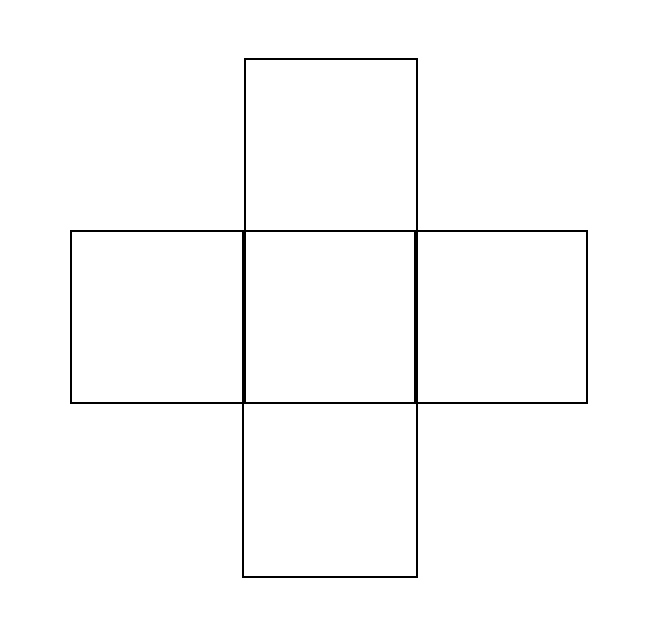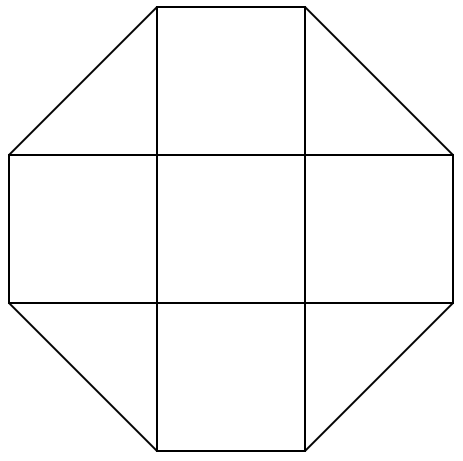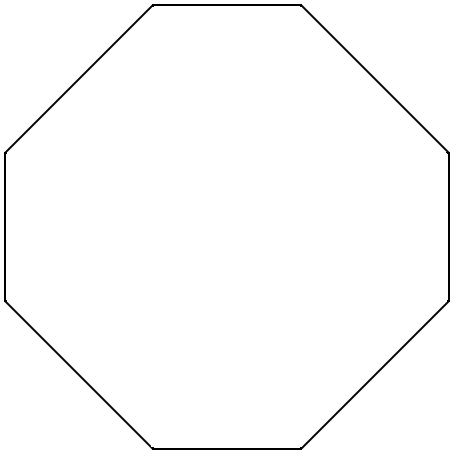# Draw an octagon using a set square with 4 right angles.Verified
90.6k+ views
Hint: In the above question, we have to draw an octagon using a set square with 4 right angles. Since we only have to use set squares, that means we can draw lines, triangles, squares, etc. In order to approach our solution, we will use the squares here.

We have to draw an octagon using a set square.
We can draw the required octagon in the several small steps below.
Step 1) Draw a small square using the set square.Step 2) Draw four more squares around the drawn square, one above the square, one below the square, and one in the left and another in the right to the first square..
A plus (+) shaped figure will be obtained as shown above in the diagram. All four squares have their one side common to the first square.
Step 3) Join the all pairs of two edges that are nearest to each other.Now we have obtained the octagonal shape. We can see the four diagonals making right angles at their point of intersections.
Step 4) Erase the lines drawn inside the octagon.That is our required octagon drawn with the help of a set square.

Note: A set square is a triangle shaped mathematical instrument used in mathematical constructions and also in technical drawings, with helps in providing a straight edge at a right angle or other particular planar angle to a baseline. In comes in a pair of $30^\circ - 60^\circ - 90^\circ$ and $45^\circ - 45^\circ - 90^\circ$ set squares.
Various other shapes other than an octagon can be drawn using a pair of set squares, like a trapezium, rectangle, square, hexagon, etc.